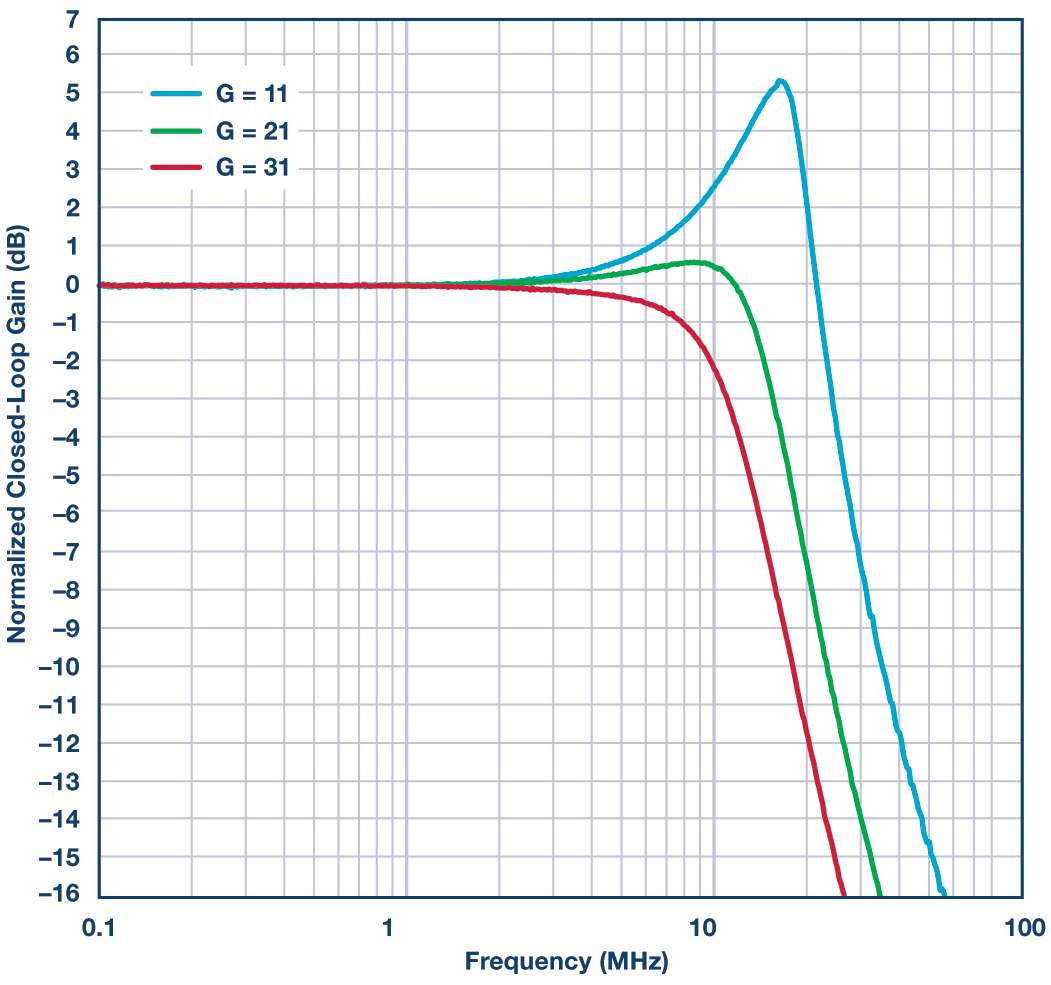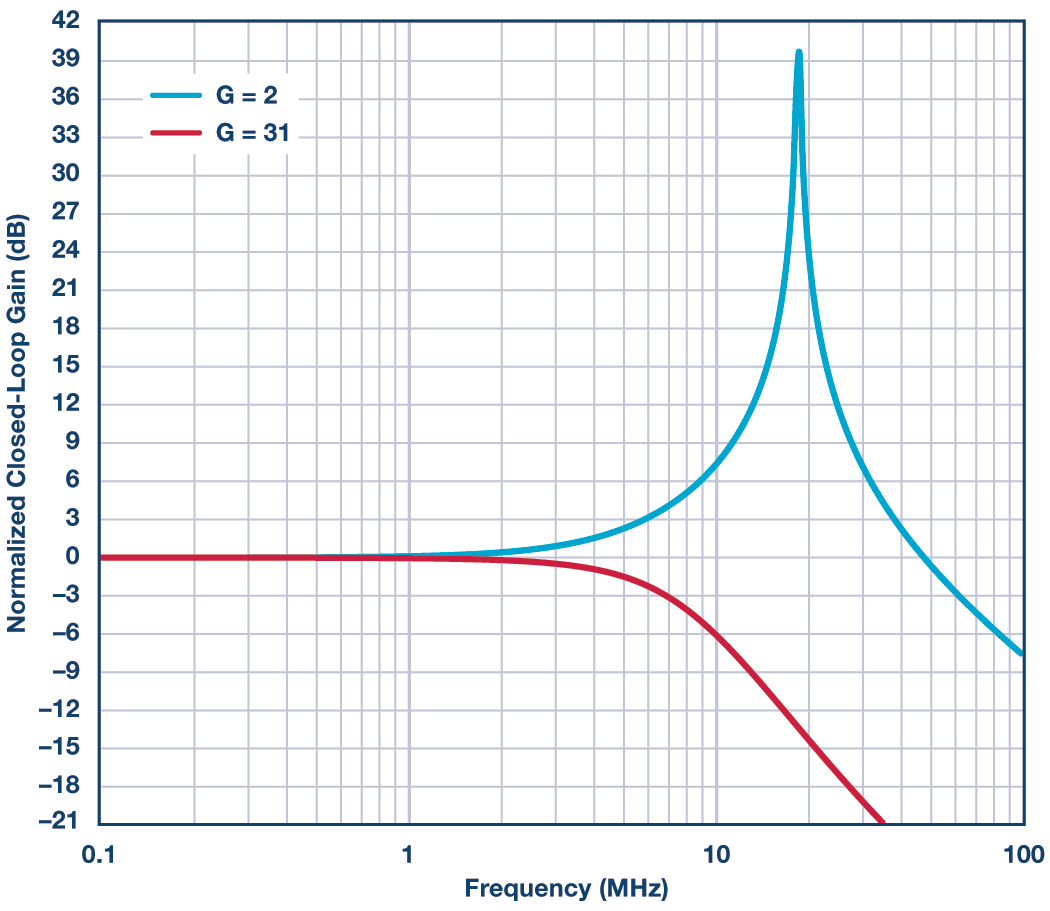# Amplifier RF: Think Before you Choose!

### QUESTION:

I’m selecting an op amp for my precision signal path. Isn’t faster always better?

When selecting the feedback resistor (RF) for single-ended voltage feedback and a fully differential amplifier, consideration needs to be given to the system requirements. There are trade-offs in the selection of RF, which include power dissipation, bandwidth, and stability. If speed is critical, as discussed in RAQ Issue 122, “The Truth About Voltage Feedback Resistors,” the recommended data sheet RF value is advised. If power dissipation is critical, and the system requires a higher gain, a larger RF can be the right choice.

The choices for RF increase as gain increases. The destabilizing effect between the internal capacitance of the amplifier and the feedback resistor is reduced with larger gains. As gain increases, the amplifier is less sensitive to gain peaking.

The example of Figure 1 shows the lab results of the normalized frequency response for the ADA4807-1, low noise, rail-to-rail input and output, voltage feedback amplifier in a noninverting configuration with an RF of 10 kΩ for gains of 11 V/V, 21 V/V, and 31 V/V.

The degree of peaking in the small signal frequency response indicates instability. Increasing the gain from 11 V/V to 31 V/V results in less than 1 dB of peaking. This would imply that the amplifier has sufficient phase margin with an RF of 10 kΩ and is stable at high gains.Figure 1. Lab results for different gains with RF = 10 kΩ. VS = ±5 V, RLOAD = 1 kΩ for gains 11 V/V, 21 V/V, and 3 V/V.Figure 2. Simulation results using the ADA4807 SPICE model. RF = 10 kΩ, VS = ±5 V, RLOAD = 1 kΩ for gain = 2, and 31 V/V.

Validating a circuit in the lab is not a mandatory step for verifying potential instabilities. Figure 2 shows the simulation results using the SPICE model with gain of 2 V/V and 31 V/V. The instability with using a large gain resistor such as 10 kΩ in a gain of 2 V/V is shown in comparison with the same RF in a gain of 31 V/V. Figure 3 shows results for gains 11 V/V, 21 V/V, and 31 V/V in the time domain.Figure 3. Pulse response simulation results using the ADA4807 SPICE model. VS = ±5 V, RF = 10 kΩ; G = 11 V/V, 21 V/V, and 31 V/V and RLOAD = 1 kΩ.

There are system trade-offs in the selection of RF. To achieve full performance from a system, the appropriate RF choice will depend on the system requirements with respect to stability, bandwidth, and power.